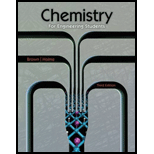Chapter 1, Problem 1.77PAE### Chemistry for Engineering Students

3rd Edition
Lawrence S. Brown + 1 other
ISBN: 9781285199023

#### Solutions

Chapter
Section### Chemistry for Engineering Students

3rd Edition
Lawrence S. Brown + 1 other
ISBN: 9781285199023
Textbook Problem

# Suppose that a new material has been devised with an elastic modulus of 22.0 x 106 psi for a bicycle frame. Is this bike frame likely to be more or less stiff than an aluminum frame?

Interpretation Introduction

Interpretation:

The stiffness of bicycle frame made from new material should be compared to the stiffness of an aluminum frame.

Concept introduction:

The stiffness of any material depends on its elasticity. Elasticity is a property of any material through which it can be stretched from its regular shape or can become flexible.

Any matter resumes its size after stretching through the help of its elasticity. The elasticity is different for different matters.

The stiffness of any material is measured in modulus which is the ratio of stress to the strain for the material as:

$E=\frac{Stress}{Strain}$

Higher the modulus more will be the stiffness of the material.

Explanation

Given:

The modulus of new material is $E=22.0×{10}^{6}psi$

Actually, higher the value of elastic modulus more will be the stiffness of the material.

Here, in given new material the elastic modulus is $22...$

### Still sussing out bartleby?

Check out a sample textbook solution.

See a sample solution

#### The Solution to Your Study Problems

Bartleby provides explanations to thousands of textbook problems written by our experts, many with advanced degrees!

Get Started

## Additional Science Solutions

#### Find more solutions based on key concepts